Special Curves
Go to   Fun_Math Content Table   Trisecting an Angle

### Trisection using Special Curves

Hippias's(about 460BC-about 400BC) Trisection method is shown in the figure shown below.The procedure for Trisection is:

Step 1:Draw the quadratrix , then select a point "A" on this curve to define angle AOB

Step 2:Draw a line from "A" parallel to AB and the intersection with line PO is point "C".

Step 3:Find a point "D" on line CO such that OD = CO/3

Step 4:Draw a line from "D" parallet to OB, and find a point "E" ,intersecting this curve.

Angle EOB trisects angle AOB.

You can see the process in animation. For detail, go to the section Quadratrix - Hippias.

#### 2. The Conchoid-invented by Nicomedes,used by Pappus

Trisection using Conchoid is shown in the figure shown below.
This curve was first called "Cochloid", then later named "Conchoid". It was invented by Nicomedes(about 280 BC- about 210 BC) to solve the "Delian Problem", but later Pappus(about 290-about 350) found out that it can be used for Angle Trisection.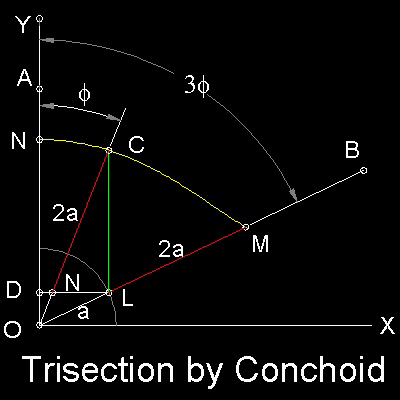The Trisection procedure is:

Step 1: Draw a rectangular coordinate Axis O-XY, and locate Point "A" on OY. Draw a line OB to define angle AOB. Angle AOB is to be trisected.

Step 2: Draw a unit radiuds(a) circle at "o", and "L" is the intersection of this circle and line OB. Draw a line from "L" parallel to OB and it intersects Y axis at point "D".

Step 3:Draw a "Conchoid" with "O" as a pole, line "DL" as "ruler" and fixed length = 2a.The resulting curve is NCM.

Step 4:Draw a line form "L" parallel to Y axis and it intersects this Conchoid at point "C". Line OC trisects angle AOB.
******** conchoid_trisection_desc.dwg ********

You can see the process in animation. For detail, go to the section Conchoid - Nicomedes.

#### 3. Archimedes' Spiral

Archimedes(287 BC - 212 BC) used Spiral (called Archimedes' Sprial) for Trisection. His idea is shown in the figure shown below.#### Trisection Steps:

1. 1.Draw Archimedian Spiral r = aq

2. 2.Select point A to define angle AOB to be trisected.

3. 3.Find intersection point "P" of line OA and this curve.

4. 4.Divide line segment OP into 3 equal parts, and let OQ = (1/3)*OP.

5. 5.Draw a circle with center at "O" and its radius OQ.

6. 6.Find point "E", which is an intersection of this circle and the curve .

7. Angle EOB = (1/3) angle AOB

************* spiral_tri_desc.dwg *************

You can see the process in animation. For detail, go to the section Spiral - Archimedes.

#### 4. The Hyperbola-Pappus

Pappus(280 - 350) showed that "Huyperbola" can be used for Angle Trisection. His idea is shown in the figure shown below.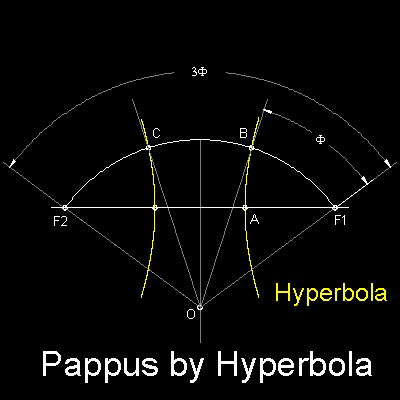The trisection of angle F1-O-F2 is equivalent to dividing arc F1-F2 into 3 equal arc length. This is accomplished by using the hyperbola with eccentricity equal to 2. It measn that for all the points on the hyperbola the distance from the F1(called Focus) is two times the normal distance to Y-axis(called Directrix). Because of symmetry the intersecting points B & C divides arc F1-O-F2 into 3 equal length arcs.
******** Pappus_hyperbola_tri_desc.dwg ********

You can see the process in animation. For detail, go to the section Hyperbola - Pappus.

#### 5. Limaçon- by Pascal

Limaçon was invented by Blaise Pascal (1623 - 1662) about 1650, and used by Isaac Newton (1643 - 1727) for Angle Trisection. The idea is shown in the figure shown below.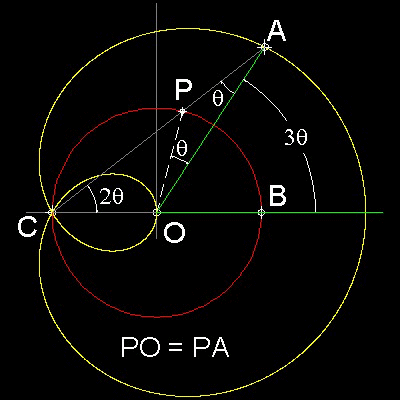##### How to draw Limaçon

Point "P" moves along a circle of unit radius CO. Let b be a fixed length. Extend CP and produce point "A" such that PA = b. Then the loucs of "A" is a Limaçon. The type of Limaçon used for Trisection is b = 1 case. The yellow colored curve is the Limason Pascal used to Trisection.

##### Trisection

Draw a unit circle with its center at "O". Draw a Limaçon with fixed length b = 1.

Select a point "A" on the Limaçon to define an angle AOB to be trisected.

Draw a line CA. Angle CAO is the angle trisected.
******** limason_tri_desc.dwg ********

You can see the process in animation. For detail, go to the section Limaçon - Pascal.

#### 6. Parabola by Rene Descartes

Rene Descartes(1596 - 1650) ,who is called the founder of the "Analytic Geometry",published the famous treatise "La Geometrie" in 1637. In this book he showed that Angle Trisection can be done by using Parabola. His idea is shown in the figure shown below.##### Trisection Procedure
1. Draw a circle with radius=2,then define a point A . angle AOB is to be trisected.

2. Drop the perpendicular from A to OB. Let the length be 2a (in Trisection equation)

3. Draw a parabola y = x2. Draw a circle with its center at point C (a, 2)

4. Get intersecting point "P", and drop the perpendicular to OB. This will cut the circle drawn at step 3 at point "T"

5. The line OT is a trisector of angle AOB.
******** parabola_tri_desc.dwg ********

You can see the process in animation. For detail, go to the section Parabola - Rene Descartes.

#### 7. The Cubic Parabola

The idea of using Parabola can be easily extended to "Cubic parabola". The idea is shown in the figure shown below.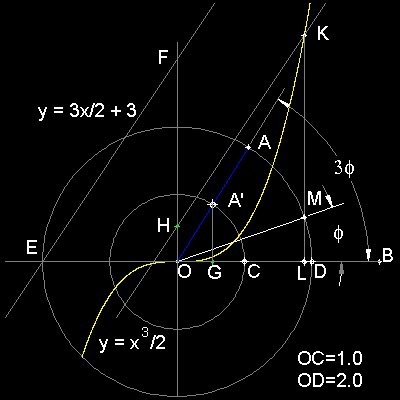##### Trisection Procedure
1. Draw the circles of radii 1 and 2. Draw a cubic parabola y = (1/2) x 3.Draw a line y = 3x/2 + 3

2. Pick a point "A" on the outer circle to define angle AOB for trisection. OA intersects the inner circle at A'. Drop a line from A'perpendicular to OB. Intersection is G. Note here that length OG is "a" in Trisection Equation.

3. Get a point H on Y-axis, such that OH = OG. Draw a line through point H parallel to line EF.

. 4. This line will cut the cubic parabola at point K. Drop a line perpendicular to OB. This intersects the inner circle at point M. Line MO is the trisector of angle AOB.

******** cubic_parabola_tri_desc.dwg ********

You can see the process in animation. For detail, go to the section Cubic Parabola.

#### 8. The Cycloid of Ceva

Tomasso Ceva(1648 - 1737) ,brother of Giovanni Ceva(1647 - 1734) ,who is known for Ceva's theorem, applied "Insertion method" by Archimedes to Angle trisection using a special curve called "Cycloidum anomalarun", or "The Cycloid of Ceva".

The idea is shown in the figure shown below.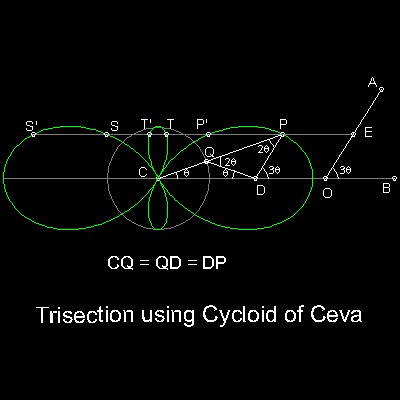##### Trisection Process
1. Draw "Cycloid of Ceva".See how to draw in animation

2. Define angle AOB

3. get unit length OE on line OA, and draw a parallel line to OB through point E.

4. This line cuts "Cycloid of Ceva" at 6 places. P,S and T are for trisectiong angles 3q , 360 deg + 3q , and 720 deg + 3q , respectively.

The remaining 3 points P', S' and T' are for 180 - 3q,
******** cycloid_of_Ceva_tri_desc.dwg ********

You can see the process in animation. For detail, go to the section Cycloidum Anomalarum - Ceva.

#### 9. Trisectrix by Maclaurin

Colin Maclaurin (1698-1746),who is known for Maclaurin Series, used a curve called "Trisectrix" to accomplish Angle Trisection.

His idea is shown in the figure shown below.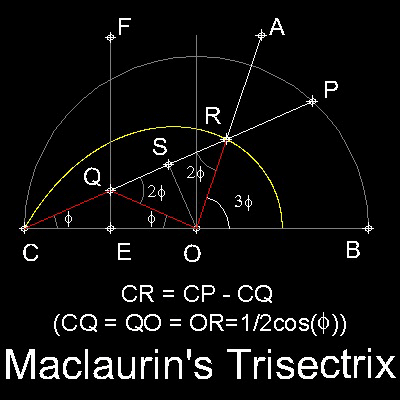##### How to draw Trisectrix
1. Draw a unit radius semi-circle BC with its center at O. EF is a perpendicular bisector of line segment CO.

2. Pick a point Q on EF, and P is the intersection of CQ and the semi_circle.

3. Locate a point R such that CR = CP - CQ is satisfied.

4. Locus of R as point Q moves along the line EF is the curve called "Maclaurin's Trisectrix".

Note: This process is also interpreted as a "imaginary" link CQ-QO-OR as Q moves along EF. It is "imaginary" because the length of the link is variable, and in reality, it does not exist.
******** Maclaurin_tri_desc.dwg ********

##### Trisection Process
This curve has the property such that angle ROB is 3 times of angle QCB. So once the curve is drawn,the trisection process is straight forward.
You can see the process in animation . For detail, go to the section Trisectrix - MacLaurin.

Go to   Fun_Math Content Table   Trisecting an Angle

All questions/suggestions should be sent to Takaya Iwamoto

Last Updated Nov 22, 2006